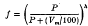Skip to main content Accessibility help
Home

# An equation to predict the leaching of surface-applied nitrate

## Summary

The fraction (f) of surface-applied nitrate leached below any depth h cm in a uniform soil profile may be calculated from the equationwhere P is the quantity of water draining through the soil (in cm) and Vm is the percentage volumetric field capacity. The fraction of nitrate retained is then (1—f).

This equation has been tested using published data. Values of h corresponding to the mean displacement (f = 0·5) were calculated for a wide range of soil and weather conditions and the results compared with mean displacements measured in the field. Similar comparisons were made with the leaching equation of Rousselle (1913) and Levin (1964). The new equation gives good agreement with the observed data, whereas the Rousselle-Levin equation generally overestimates the mean displacement of nitrate. Methods of applying the equations to field situations are discussed.

Hide All

## Metrics

### Full text viewsFull text views reflects the number of PDF downloads, PDFs sent to Google Drive, Dropbox and Kindle and HTML full text views.

Total number of HTML views: 0
Total number of PDF views: 0 *Loading metrics...

### Abstract viewsAbstract views reflect the number of visits to the article landing page.

Total abstract views: 0 *Loading metrics...

* Views captured on Cambridge Core between <date>. This data will be updated every 24 hours.

Usage data cannot currently be displayed